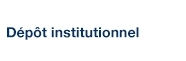# User-Base Station Association in HetSNets: Complexity and Efficient Algorithms

Mlika, Zoubeir; Goonewardena, Mathew; Ajib, Wessam et Elbiaze, Halima (2016). « User-Base Station Association in HetSNets: Complexity and Efficient Algorithms ». IEEE Transactions on Vehicular Technology(99).

Fichier(s) associé(s) à ce document :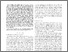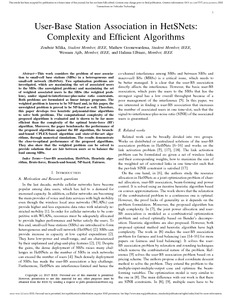Prévisualisation
PDF
Télécharger (1MB)

## Résumé

This work considers the problem of user association to small-cell base stations (SBSs) in a heterogeneous and small-cell network (HetSNet). Two optimization problems are investigated, which are maximizing the set of associated users to the SBSs (the unweighted problem) and maximizing the set of weighted associated users to the SBSs (the weighted problem), under signal-to-interference-plus-noise ratio constraints. Both problems are formulated as linear integer programs. The weighted problem is known to be NP-hard and, in this paper, the unweighted problem is proved to be NP-hard as well. Therefore, this paper develops two heuristic polynomial-time algorithms to solve both problems. The computational complexity of the proposed algorithms is evaluated and is shown to be far more efficient than the complexity of the optimal brute-force (BF) algorithm. Moreover, the paper benchmarks the performance of the proposed algorithms against the BF algorithm, the branchand- bound CPLEX-based algorithm and state-of-the-art algorithms, through numerical simulations. The results demonstrate the close-to-optimal performance of the proposed algorithms. They also show that the weighted problem can be solved to provide solutions that are fair between users or to balance the load among SBSs.

Type: Article de revue scientifique Branch-and-bound, Brute-force. Fairness, HetSNets, Heuristic algorithm, NP-hard, User-BS association Faculté des sciences > Département d'informatique Wessam Ajib 09 mai 2016 14:34 30 mai 2016 14:41 http://archipel.uqam.ca/id/eprint/8422

## Statistiques

Voir les statistiques sur cinq ans...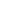RECHERCHER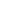PARCOURIR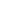LIBRE ACCÈS